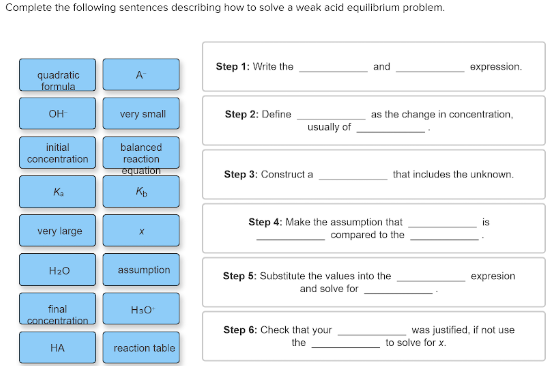# Complete the following sentences describing how to solve a weak acid equilibrium problem. Step 1: Write the ___ and ___ expression. Step 2: Define ___ as the change in concentration, usually of ___. Step 3: Construct a ___ that includes the unknown. Step 4: Make the assumption that ___ is ___ compared to the ___. Step 5: Substitute the values into the ___ expression and solve for ___. Step 6: Check that your ___ was justified, if not use the ___ to solve for x. quadratic formula, A-, OH-, very small, initial concentration, balanced reaction equation, Ka, Kb, very large, x, H2O, assumption, final concentration, H3O-, HA, reaction rate